#Interactive Real Analysis

Next | Previous | Glossary | Map

## 6.5. Differentiable Functions

### Examples 6.5.10(c):

Use the Mean Value theorem to show that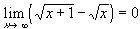Define the function f(x) =. Then f is continuous on [0,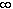) and differentiable on (0,). By the Mean Value theorem there exists a number c such that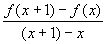= f'(c)
for c between x and x + 1. But then
0 <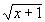-= 1/2 * 1 /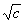As x goes to infinity, so does c (it is always bigger than x). The left side of this equation goes to 0 as c goes to infinity. Therefore, the right side must also go to zero.
Next | Previous | Glossary | Map